# Section 1 Problem Section 2 Experiment Design Section

• Slides: 11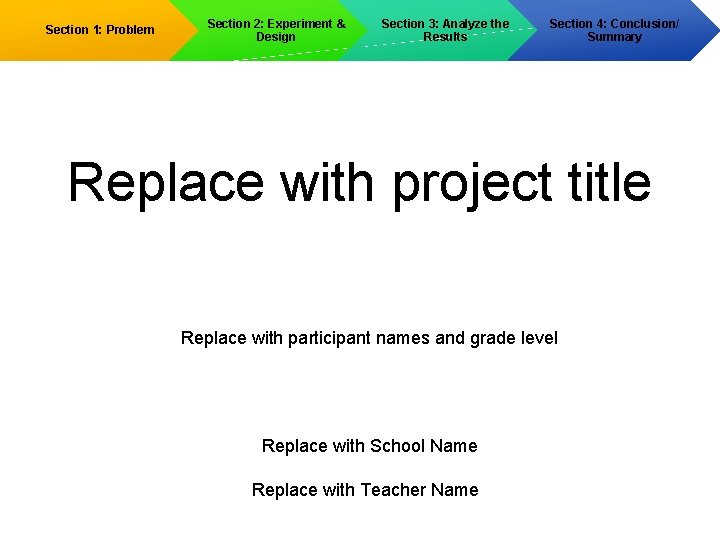Section 1: Problem Section 2: Experiment & Design Section 3: Analyze the Results Section 4: Conclusion/ Summary Replace with project title Replace with participant names and grade level Replace with School Name Replace with Teacher Name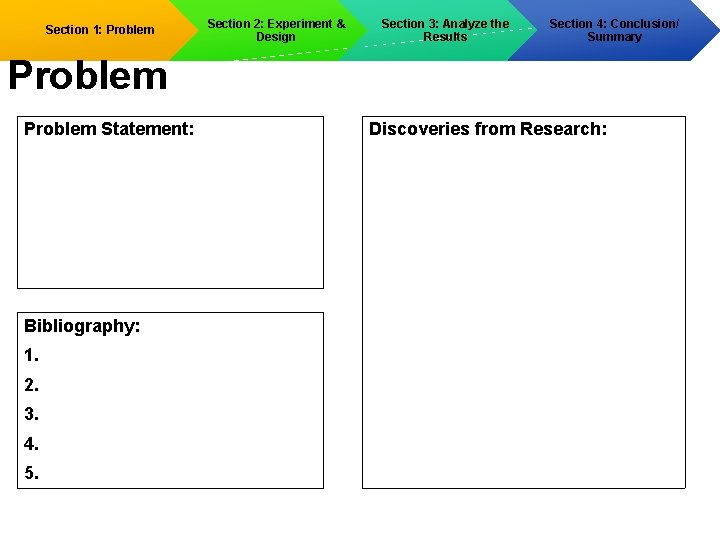Section 1: Problem Section 2: Experiment & Design Section 3: Analyze the Results Section 4: Conclusion/ Summary Problem Statement: Bibliography: 1. 2. 3. 4. 5. Discoveries from Research: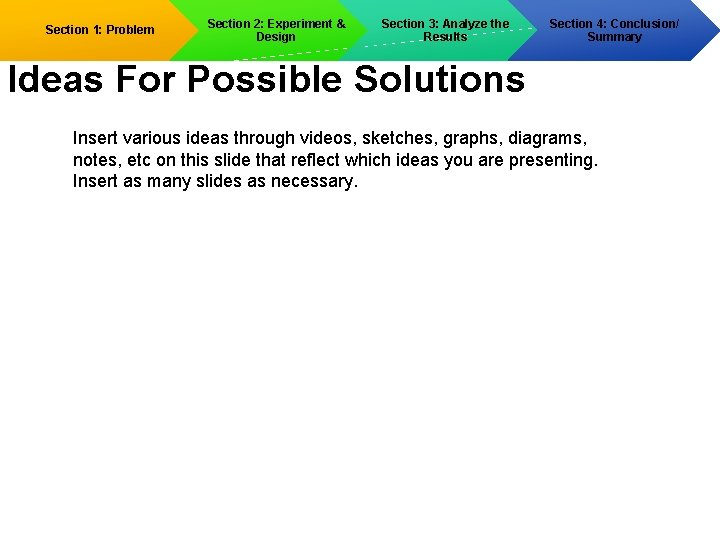Section 1: Problem Section 2: Experiment & Design Section 3: Analyze the Results Section 4: Conclusion/ Summary Ideas For Possible Solutions Insert various ideas through videos, sketches, graphs, diagrams, notes, etc on this slide that reflect which ideas you are presenting. Insert as many slides as necessary.Section 1: Problem Section 2: Experiment & Design Section 3: Analyze the Results Select A Promising Solution Narrow down the results from your research to 2 solutions. Provide information about each idea. Solution 1: Solution 2: Section 4: Conclusion/ Summary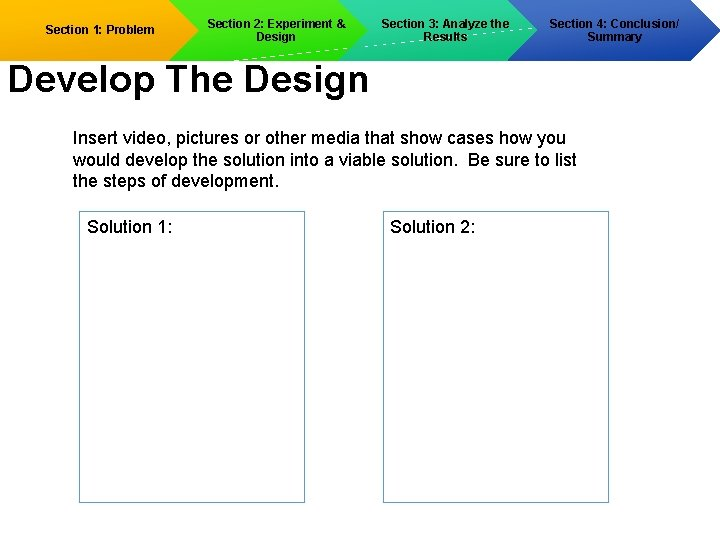Section 1: Problem Section 2: Experiment & Design Section 3: Analyze the Results Section 4: Conclusion/ Summary Develop The Design Insert video, pictures or other media that show cases how you would develop the solution into a viable solution. Be sure to list the steps of development. Solution 1: Solution 2:Section 1: Problem Section 2: Experiment & Design Section 3: Analyze the Results Section 4: Conclusion/ Summary Select a Promising Solution Cost Analysis Solution 1 Material Quantity Cost Analysis Solution 2 Source Material Quantity Cost SourceSection 1: Problem Section 2: Experiment & Design Section 3: Analyze the Results Solution Development Solution 1 Pros Cons Section 4: Conclusion/ Summary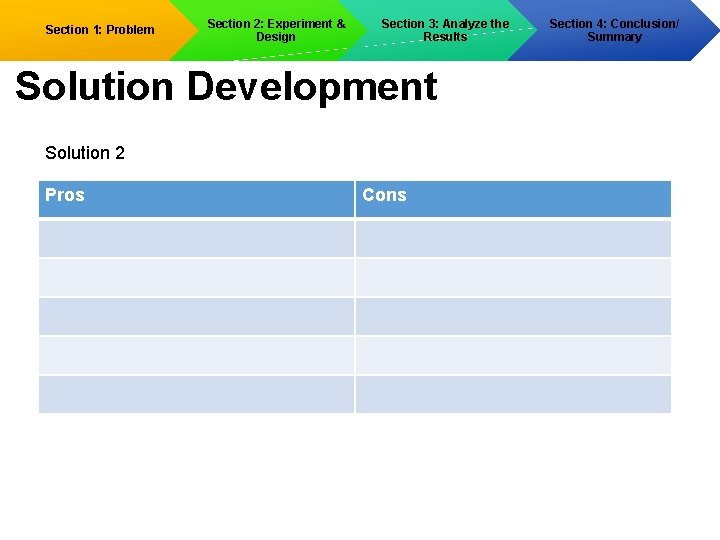Section 1: Problem Section 2: Experiment & Design Section 3: Analyze the Results Solution Development Solution 2 Pros Cons Section 4: Conclusion/ Summary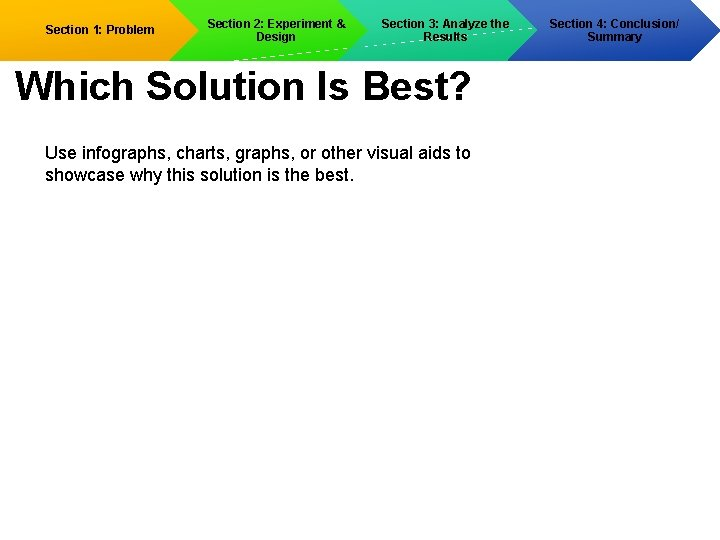Section 1: Problem Section 2: Experiment & Design Section 3: Analyze the Results Which Solution Is Best? Use infographs, charts, graphs, or other visual aids to showcase why this solution is the best. Section 4: Conclusion/ Summary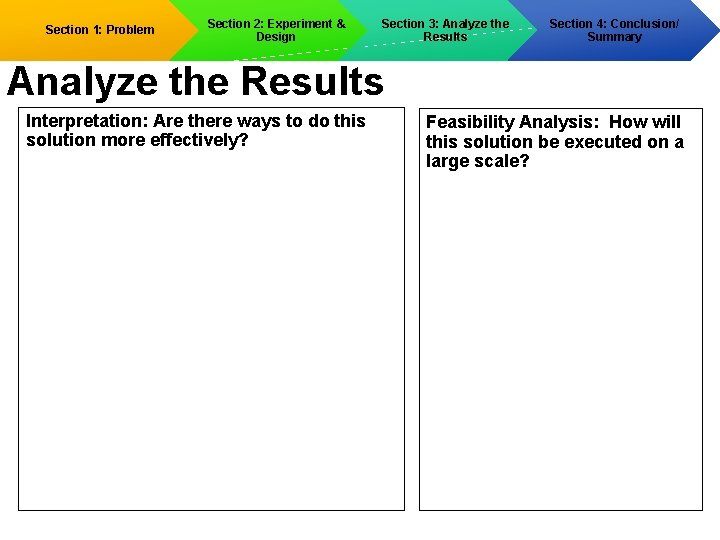Section 1: Problem Section 2: Experiment & Design Section 3: Analyze the Results Section 4: Conclusion/ Summary Analyze the Results Interpretation: Are there ways to do this solution more effectively? Feasibility Analysis: How will this solution be executed on a large scale?Section 1: Problem Section 2: Experiment & Design Section 3: Analyze the Results Section 4: Conclusion/ Summary & Conclusion Problem statement Implementation summary Data Summary How will this solution solve the problem?